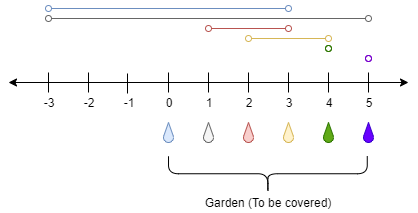1326. Minimum Number of Taps to Open to Water a Garden

There is a one-dimensional garden on the x-axis. The garden starts at the point `0` and ends at the point `n`. (i.e The length of the garden is `n`).

There are `n + 1` taps located at points `[0, 1, ..., n]` in the garden.

Given an integer `n` and an integer array `ranges` of length `n + 1` where `ranges[i]` (0-indexed) means the `i-th` tap can water the area `[i - ranges[i], i + ranges[i]]` if it was open.

Return the minimum number of taps that should be open to water the whole garden, If the garden cannot be watered return -1.

Example 1:```Input: n = 5, ranges = [3,4,1,1,0,0]
Output: 1
Explanation: The tap at point 0 can cover the interval [-3,3]
The tap at point 1 can cover the interval [-3,5]
The tap at point 2 can cover the interval [1,3]
The tap at point 3 can cover the interval [2,4]
The tap at point 4 can cover the interval [4,4]
The tap at point 5 can cover the interval [5,5]
Opening Only the second tap will water the whole garden [0,5]
```

Example 2:

```Input: n = 3, ranges = [0,0,0,0]
Output: -1
Explanation: Even if you activate all the four taps you cannot water the whole garden.
```

Example 3:

```Input: n = 7, ranges = [1,2,1,0,2,1,0,1]
Output: 3
```

Example 4:

```Input: n = 8, ranges = [4,0,0,0,0,0,0,0,4]
Output: 2
```

Example 5:

```Input: n = 8, ranges = [4,0,0,0,4,0,0,0,4]
Output: 1
```

Constraints:

• `1 <= n <= 10^4`
• `ranges.length == n + 1`
• `0 <= ranges[i] <= 100`

1326. Minimum Number of Taps to Open to Water a Garden
``````struct Solution;

impl Solution {
fn min_taps(n: i32, ranges: Vec<i32>) -> i32 {
let n = n as usize;
let mut jumps = vec![0; n + 1];
for i in 0..=n {
let d = ranges[i];
let l = 0.max(i as i32 - d) as usize;
let r = n.min(i + d as usize);
jumps[l] = jumps[l].max(r - l);
}
let mut end = 0;
let mut reach = 0;
let mut res = 0;
for i in 0..n {
if i > reach {
return -1;
}
reach = reach.max(i + jumps[i]);
if i == end {
res += 1;
end = reach;
}
}
if reach >= n as usize {
res
} else {
-1
}
}
}

#[test]
fn test() {
let n = 5;
let ranges = vec![3, 4, 1, 1, 0, 0];
let res = 1;
assert_eq!(Solution::min_taps(n, ranges), res);
let n = 3;
let ranges = vec![0, 0, 0, 0];
let res = -1;
assert_eq!(Solution::min_taps(n, ranges), res);
let n = 7;
let ranges = vec![1, 2, 1, 0, 2, 1, 0, 1];
let res = 3;
assert_eq!(Solution::min_taps(n, ranges), res);
let n = 8;
let ranges = vec![4, 0, 0, 0, 0, 0, 0, 0, 4];
let res = 2;
assert_eq!(Solution::min_taps(n, ranges), res);
}
``````